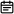# 70. 爬楼梯2019-06-21

## 题目链接

https://leetcode-cn.com/problems/climbing-stairs/

## 题目描述

输入： 2

1.  1 阶 + 1 阶
2.  2 阶

输入： 3

1.  1 阶 + 1 阶 + 1 阶
2.  1 阶 + 2 阶
3.  2 阶 + 1 阶

## 解题方案

### 第一种思路

• 标签：数学
• 如果观察数学规律，可知本题是斐波那契数列，那么用斐波那契数列的公式即可解决问题，公式如下：

$F_n = 1/\sqrt{5}\Big[\Big(\frac{1+\sqrt{5}}{2}\Big)^n-\Big(\frac{1-\sqrt{5}}{2}\Big)^n\Big]$

• 时间复杂度：O(logn)

### 第一种思路代码

• Java版本
class Solution {
public int climbStairs(int n) {
double sqrt_5 = Math.sqrt(5);
double fib_n = Math.pow((1 + sqrt_5) / 2, n + 1) - Math.pow((1 - sqrt_5) / 2,n + 1);
return (int)(fib_n / sqrt_5);
}
}
• JavaScript版本
/**
* @param {number} n
* @return {number}
*/
var climbStairs = function(n) {
const sqrt_5 = Math.sqrt(5);
const fib_n = Math.pow((1 + sqrt_5) / 2, n + 1) - Math.pow((1 - sqrt_5) / 2,n + 1);
return Math.round(fib_n / sqrt_5);
};

### 第二种思路

• 标签：动态规划
• 本问题其实常规解法可以分成多个子问题，爬第n阶楼梯的方法数量，等于2部分之和
• 爬上n-1阶楼梯的方法数量。因为再爬1阶就能到第n阶
• 爬上n-2阶楼梯的方法数量，因为再爬2阶就能到第n阶
• 所以我们得到公式dp[n] = dp[n-1] + dp[n-2]
• 同时需要初始化dp=1dp=1
• 时间复杂度：O(n)

### 第二种思路代码

• Java版本
class Solution {
public int climbStairs(int n) {
int[] dp = new int[n + 1];
dp = 1;
dp = 1;
for(int i = 2; i <= n; i++) {
dp[i] = dp[i - 1] + dp[i - 2];
}
return dp[n];
}
}
• JavaScript版本
/**
* @param {number} n
* @return {number}
*/
var climbStairs = function(n) {
const dp = [];
dp = 1;
dp = 1;
for(let i = 2; i <= n; i++) {
dp[i] = dp[i - 1] + dp[i - 2];
}
return dp[n];
};

### 画解